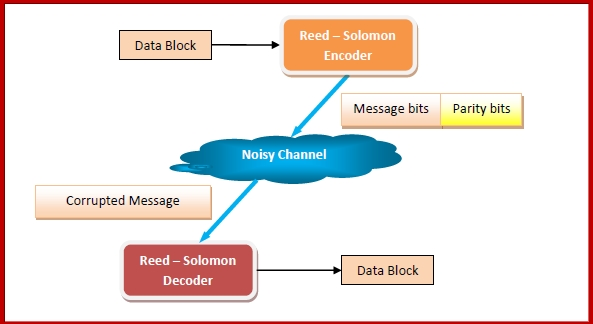# Error Correcting Codes - Reed-Solomon codes

## Errors and Error Correcting Codes

Errors in data occur when bits get corrupted in the data. When bits are transmitted over the computer network, they are subject to get corrupted due to interference and network problems, leading to errors.

Error-correcting codes (ECC) are a sequence of numbers generated by specific algorithms for detecting and removing errors in data that has been transmitted over noisy channels. Error correcting codes ascertain the exact number of bits that has been corrupted as well as the location of the corrupted bits, within the limitations in algorithm.

ECCs can be broadly categorized into two types, block codes and convolution codes. Reed - Solomon Code is a type of block code.

## Reed - Solomon Code

Reed - Solomon error correcting codes are one of the oldest codes that were introduced in 1960s by Irving S. Reed and Gustave Solomon. It is a subclass of non - binary BCH codes.  BCH codes (Bose-Chaudhuri-Hocquenghem codes) are cyclic ECCs that are constructed using polynomials over data blocks.

A Reed - Solomon encoder accepts a block of data and adds redundant bits (parity bits) before transmitting it over noisy channels. On receiving the data, a decoder corrects the error depending upon the code characteristics.## Application Areas of Reed-Solomon Codes

The prominent application areas are −

• Storage areas like CDs, DVDs, Blu-ray Discs

• High speed data transmission technologies such as DSL and WiMAX

• High speed modems

• QR Codes

• Broadcast systems such as satellite communications

• Storage systems such as RAID 6

## Parameters of Reed - Solomon Codes

• A Reed-Solomon code is specified as RS(n,k).

• Here, n is the block length which is recognizable by symbols, holding the relation, n = 2m - 1.

• The message size is of k bits.

• So the parity check size is (n - k) bits

• The code can correct up to (t) errors in a codeword, where (2t = n - k).

The following diagram shows a Reed-Solomon codeword −## Generator Polynomial of Reed Solomon Code

In coding systems with block codes, valid code words consists of polynomials that are divisible by another fixed polynomial of short length. This fixed polynomial is called generator polynomial.

In Reed Solomon code, generator polynomial with factors is constructed where each root is a consecutive element in the Galois field. The polynomial is of the form −

g(x) = (x - α) (x - α2) (x - α3) ......(x - α2t)where α is a primitive element.

## Encoding using Reed Solomon Code

The method of encoding in Reed Solomon code has the following steps −

• The message is represented as a polynomial p(x), and then multiplied with the generator polynomial g(x).

• The message vector [x1,x2,x3.....xk] is mapped to a polynomial of degree less than k such that pxi) = xi for all i = 1,...k

• The polynomial is evaluated using interpolation methods like Lagrange Interpolation.

• Using this polynomial, the other points αk + 1....αn, are evaluated.

• The encoded message is calculated as s(x) = p(x) * g(x). The sender sends this encoded message along with the generator polynomial g(x).

## Decoding using Reed Solomon Code

At the receiving end, the following decoding procedure done −

• The receiver receives the message r(x) and divides it by the generator polynomial g(x).

• If r(x)/g(x)=0, then it implies no error.

• If r(x)/g(x)≠0, then the error polynomial is evaluated using the expression: r(x) = p(x) * g(x) + e(x)

• The error polynomial gives the error positions.